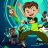# Bollinger Band Contraction/Expansion

H

#### Happymono

##### New member
VIP
Looked around to see if there were any scripts similar but to no avail the idea being that the color will shift in regards to the bands contracting or expanding
also curious if the color shift could be plotted onto the middle bollinger moving average to lessen the amount of lower indicatorsCode:
``````//@version=3
// Hector R. Madrid : Bollinger Bands Width.  : 6/JUN/2014 22:57 : 2.0
// The contractions and expansions of the bands are
// represented by two different colors.
//

src = input(close, type=source)
length = input(34, minval=1), mult = input(2.0, minval=0.001, maxval=50)

basis = sma(src, length)
dev = stdev(src, length)
upper1 = basis + dev
lower1 = basis - dev
upper2 = basis + 2*dev
lower2 = basis - 2*dev

bbw = (upper2 - lower2)*100/lower2

// Output
plot(bbw, color=bbw-ema(bbw,5)>=0?teal:navy, linewidth=2, style=area)``````#### BenTen

Staff
VIP
Check out the existing BollingerBandwidth indicator in ThinkorSwim.

Code:
``````#
# TD Ameritrade IP Company, Inc. (c) 2008-2020
#

declare lower;

input averageType = AverageType.Simple;
input price = close;
input displace = 0;
input length = 20;
input Num_Dev_Dn = -2.0;
input Num_Dev_Up = 2.0;
input BulgeLength = 150;
input SqueezeLength = 150;

def upperBand = BollingerBands(price, displace, length, Num_Dev_Dn, Num_Dev_Up, averageType).UpperBand;
def lowerBand = BollingerBands(price, displace, length, Num_Dev_Dn, Num_Dev_Up, averageType).LowerBand;
def midLine = BollingerBands(price, displace, length, Num_Dev_Dn, Num_Dev_Up, averageType).MidLine;

plot Bandwidth = (upperBand - lowerBand) / midLine * 100;
Bandwidth.SetDefaultColor(GetColor(1));

plot Bulge = Highest(Bandwidth, BulgeLength);
Bulge.SetDefaultColor(GetColor(8));
Bulge.setStyle(Curve.SHORT_DASH);

plot Squeeze = Lowest(Bandwidth, SqueezeLength);
Squeeze.SetDefaultColor(GetColor(8));
Squeeze.setStyle(Curve.SHORT_DASH);``````

H

#### Happymono

##### New member
VIP
Thank you for the suggestion though i'm embarrassed to say i'm no pro at reading that default and was why i looked into visual green/red light sort of band width indicatorBollinger Band Stops Indicator for ThinkorSwim Custom 10